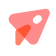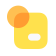# 比亚迪宋Plus EV

30人加入

•发动态
•发文章
•发点评
{each \$index as \$item}
{if [1, 2, 4, 7, 16, 25].indexOf(\$item.type) != -1}
{if style === 'follow'}

{\$item.data.create_time}

{else if style === 'home'} {else} {/if}
{if \$item.type == 1} {if \$item.data.category_id == 11} {else}

## {\$item.data.title}

{/if} {/if} {if \$item.type == 2}

## {\$item.data.title}

{\$item.data.summary}

{\$item.data.duration} {if \$item.data.PlayURL} {else} {/if}

{/if} {if \$item.type == 4}

{if \$item.data.video}

{/if} {if \$item.data.imgs_url.length}

{each \$item.data.imgs_url as \$img_url} {if \$img_url.path}{/if} {/each}

{/if}
{/if} {if \$item.type == 7}

{\$item.data.title}

{each \$item.data.options as \$option} {set \$percent = (\$item.data.votenum)?round(\$option.number/\$item.data.votenum*100):0}
• {\$option.name} {\$option.number}
• {/each}
{/if} {if \$item.type == 16}

### {\$item.data.series.name} {(\$item.data.model) ? \$item.data.model.name : ''}

{if \$item.data.contents.attachment.length}

{each \$item.data.contents.attachment as \$att}{/each}

{/if}
{/if} {if \$item.type == 25}

## {\$item.data.title}

{/if} {if \$item.data.circle === undefined}{if \$item.data.related.length}

{each \$item.data.related as \$related} {if \$related.type == 29} {\$related.circle.title} {/if} {if \$related.type == 30} {\$related.theme.title} {/if} {/each}

{/if}{/if}
{/if} {if \$item.type == 27}
{/if} {if \$item.type == 29}## {\$item.data.title}{\$item.data.membernum}人参与讨论

{/if} {if \$item.type == 30}{/if}
{/each}

## 发布动态

• ### 添加图片

最多可以添加 9 张图片，直接拖动图片可调整顺序，双击设为封面
• ### 添加视频

请上传5分钟以内的视频，请勿上传色情、反动等违法视频

视频上传中

## 发布点评

请选择车型
• ### 添加图片

最多可以添加 9 张图片，直接拖动图片可调整顺序，双击设为封面
{each Object.keys(\$allBrand) as \$initial}
• {\$initial}
• {/each}
{each \$allBrand as \$list \$initial} {each \$list as \$brand}
•{\$brand.name}
{each \$brand.series as \$manufacturer} {if \$brand.series.length}
{\$manufacturer.name}
{/if} {each \$manufacturer.list as \$series}
{\$series.name}
{/each} {/each}
• {/each} {/each}